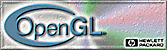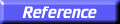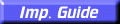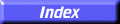# glOrtho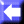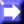glOrtho: multiply the current matrix with an orthographic matrix.

C Specification | Parameters | Description | Errors | Associated Gets | See Also

##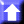C Specification

```void glOrtho(
GLdouble	 left,
GLdouble	 right,
GLdouble	 bottom,
GLdouble	 top,
GLdouble	 zNear,
GLdouble	 zFar)
```

##Parameters

left, right
Specify the coordinates for the left and right vertical clipping planes.
bottom, top
Specify the coordinates for the bottom and top horizontal clipping planes.
zNear, zFar
Specify the distances to the nearer and farther depth clipping planes. These values are negative if the plane is to be behind the viewer.

##Description

glOrtho describes a transformation that produces a parallel projection. The current matrix (see glMatrixMode) is multiplied by this matrix and the result replaces the current matrix, as if glMultMatrix were called with the following matrix as its argument:

 A 0 0 tx 0 B 0 ty 0 0 C tz 0 0 0 1

where

A =
2 / (right
left)
B =
2 / (topbottom)
C =2 / (farnear)
tx =(right + left) / (rightleft)
ty =(top + bottom) / (topbottom)
tz =(zFar + zNear) / (zFarzNear)

Typically, the matrix mode is GL_PROJECTION, and (left, bottom,zNear) and (right, top,zNear) specify the points on the near clipping plane that are mapped to the lower left and upper right corners of the window, respectively, assuming that the eye is located at (0, 0, 0).zFar specifies the location of the far clipping plane. Both zNear and zFar can be either positive or negative.

Use glPushMatrix and glPopMatrix to save and restore the current matrix stack.

##Errors

• GL_INVALID_OPERATION is generated if glOrtho is executed between the execution of glBegin and the corresponding execution of glEnd.

##Associated Gets

glGet with argument GL_MATRIX_MODE
glGet with argument GL_MODELVIEW_MATRIX
glGet with argument GL_PROJECTION_MATRIX
glGet with argument GL_TEXTURE_MATRIX

glFrustum,
glMatrixMode,
glMultMatrix,
glPushMatrix,
glViewport